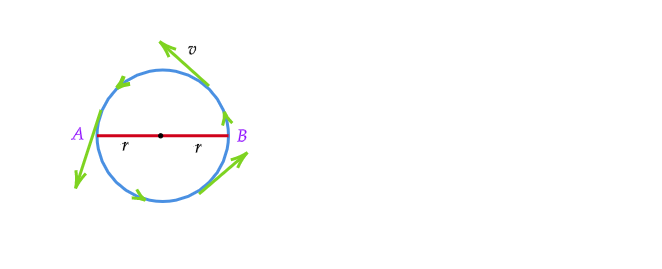# A body is moving along a circular path of radius (r) with uniform speed (v) n1 . What is the direction of instantaneous velocity? n2. What is magnitude of average velocity in (I) half rotation and (ii) three fourth rotationn3. What is the direction of instantaneous acceleration?

1. Direction of the instantaneous velocity of the particle will be in the direction of the tangent to the circular path.

2.Here given, the radius of the circular path $=r$

Therefore, the length of the half-circular path $=\pi r$

Velocity of the moving particle $=v$

Therefore, time taken by the particle to reach form B to A $=\frac{\pi r}{v}$

We know, displacement is $=r+r=2r$

Therefore, average velocity $=\frac{total\ displacement}{time}$

$=\frac{2r}{\frac{\pi r}{v}}$

$=\frac{2vr}{\pi r}$

$=\frac{2v}{\pi}$

3. Direction of the acceleration of the particle will be in the direction of the tangents to the circular path.